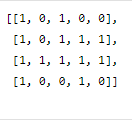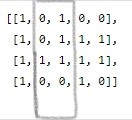# Largest Submatrix with Equal Number of 0's and 1's

Posted: 2 Jan, 2021
Difficulty: Easy

## PROBLEM STATEMENT

#### For example, for the following grid:``````The largest sub-matrix having an equal number of 0s and 1s in the above grid is shown below :
````````````The area of the largest sub-matrix having an equal number of 0s and 1s is 4(rows in highlighted image) * 2(columns in highlighted image) = 8.
``````
##### Input Format:
``````The first line of input contains an integer ‘T’ denoting the number of test cases. Then each test case follows.

The first line of each test case contains two single space-separated integers ‘N’ and ‘M’ representing the number of rows and columns of the grid, respectively.

The next 'N' lines of each test case contain 'M' single space-separated integers 0 or 1.
``````
##### Output Format:
``````For each test case, print the area of the largest possible sub-matrix having an equal number of 0s and 1s.

Print the output of each test case in a separate line.
``````
##### Note
``````You don’t have to print anything, it has already been taken care of. Just complete the function.
``````
##### Constraints:
``````1 <= T <= 10^2
1 <= N <= 50
1 <= M <= 50
MAT[i][j] = 0 or 1

Time Limit: 1 sec
``````Approach 1

We will naively count 0s and 1s in all possible submatrices and return the area of the largest submatrix having an equal number of 0s and 1s.